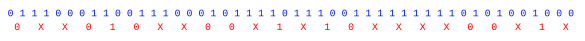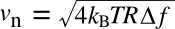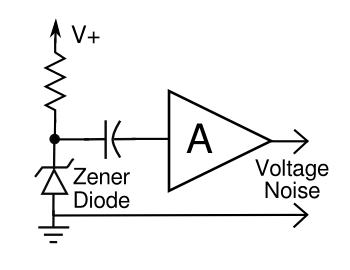### Casting Lots in the Digital Age

June 23, 2016

Random numbers are an important part of computer programming. Aside from their obvious use as a method of throwing "digital dice" for computer versions of board games and generating random play in other computer games, they're used extensively in scientific simulations. One of the earliest uses of random numbers in computer simulation was for the Monte Carlo method, developed principally by Nicholas Metropolis in the early 1950s.

John von Neumann (1903-1957), a founding figure in computing, was involved also with the development of the Monte Carlo method. Von Neumann was most concerned that the numbers generated by a computer were as close to random as possible.

Since these "random" numbers are actually produced by an algorithm, they aren't really random, just pseudorandom. Von Neumann cautioned against taking the pseudorandom character of these numbers too lightly when he said, "Any one who considers arithmetical methods of producing random digits is, of course, in a state of sin."

Von Neumann advanced pseudorandom number generation in two ways. First, he invented the middle-square method, a rapid generator suitable for simple applications. An example of this method for generating four digit random numbers follows:
• Select a four-digit "seed" number: 5678
Square the number: 32239684
Pad the number to the left with zeros if it's less than eight digits.
• Select the middle four digits: 2396
• This is our first random number, and the seed for the next random number.

In spreadsheet programs, such as Gnumeric, you can select the middle digits of a number n using the modulus and integer functions; i.e., =mod(int(n/100),10000). Spreadsheets have their own random function, which is better to use. The first few subsequent numbers generated in the above example are 7408, 8784, 1586, 5153, 5534, 6251, 750, 5625, 6406, and 368. There are two problems with von Neumann's middle-square method. If the middle digits become all zeros, subsequent output is always zero. The generator can also enter a mode that outputs the same short sequence, again, and again.

Von Neumann further created a process to extract a greater degree of randomness from a biased coin toss. Since there are two states (head/tail) in a coin toss, this Von Neumann Whitening is simply applied to a bitstream of ones and zeros. The method takes two successive bits at a time, and maps them to a random stream as follows: (0,1) → 0, (1,0) → 1, (0,0) → NULL, (1,1) → NULL, the NULL meaning that the result is ignored, and you move on to the next two bits of input data. As can be seen in the example below of a bitstream slightly biased to 1, this method discards a lot of bits.Von Neumann whitening (randomness extractor) applied to a slightly biased random bitstream. (Drawn using Inkscape.)

A simple random bit generator, implemented easily in either hardware or software, is the linear feedback shift register. This is just a shift register with its input derived from a combination of its contained bits. A 16-bit version having feedback from bits 4, 13, 15, and 16, is shown in the figure. A 32-bit register would have taps at bits 1, 2, 22, and 32, while a 64-bit register would have taps at 60, 61, 63, and 64.16-bit maximal length (65,535) linear feedback shift register (LFSR) random bit generator. In this example, exclusive-or (XOR) gates are used to effect the feedback function. (Drawn using Inkscape.)

The 16-bit pseudorandom number generator shown above will give you 65,535 bits before repeating, and you can extract as many 16-bit numbers by taking data from the register bits. Another simple random number generator is the linear congruential generator, a recurrence relation published in 1948 by D.H. Lehmer. I wrote about this generator in an earlier article (One Time Pads, June 12, 2013).

These random number generators can be sufficient for simple simulations, but anything so predictable is not suitable for cryptography. That's why more advanced pseudorandom number generators, such as the Mersenne Twister, have been developed. As its name implies, the Mersenne Twister is based on the idea of the Mersenne primes, and its 32-bit implementation, called MT19937, has a period 219937 - 1. It passes the stringent Diehard suite of statistical tests for randomness.

Since algorithmic sources of randomness are always problematic, you can look to nature to give you some physical sources of randomness. In 1926, John B. Johnson of Bell Labs discovered that resistors produce a noise voltage. His Bell Labs colleague, Harry Nyquist, was able to explain this phenomenon, which is now known as Johnson-Nyquist noise.

This random voltage noise exists across any resistor at any temperature above absolute zero. Its value is,where vn is the root-mean-square (rms) noise voltage, kB is the Boltzmann constant (1.3806 x 10−23 joule/kelvin), R is the resistance (ohms), T is the absolute temperature (kelvin), and Δf is the frequency interval over which the noise voltage is measured (hertz). Since the noise voltage doesn't depend on where the frequency interval is taken, it's frequency-independent noise; that is, "white noise."

The amplitude of Johnson-Nyquist noise is small, so electrical engineers have found better noise sources. Although the primary purpose of a Zener diode is to act as a voltage reference or voltage limiter, a reverse-biased Zener diode will produce a noise voltage. A basic circuit is shown in the figure, and two amplifiers are usually cascaded to produce volt-level signals. Zener diodes with higher breakdown voltage produce stronger noise signals.White noise voltage generator using a reverse-biased Zener diode.

This analog noise can be converted into a digital bitstream using additional circuitry.

(Drawn using Inkscape.)

There are various other ways to generate random signals using electronics, the technique in ref. 6 being one example. Physical processes that are definitely random, in the sense of "God playing dice with the world" random, are quantum processes such as radioactive decay. I wrote about one method of extracting quantum randomness in an earlier article (Quantum Random Numbers, September 27, 2010).If God does play dice with the world, how many faces would his dice have?

Some physicists think the number might be 10 or 11.

(Wikimedia Commons image by Clément Bucco-Lechat.)

Computer scientists are still working to improve random number generation. Recently, computer scientists at the University of Texas at Austin (Austin, Texas) have published a method that allows the combination of two data streams of weak randomness into a truly random data stream.[8-9] Their randomness extractor is a huge advance over Von Neumann Whitening, it's not too computationally demanding, and it's won acclaim from other researchers.

University of Texas at Austin computer science professor, David Zuckerman, and his graduate student, Eshan Chattopadhyay, have posted a draft of their work on the Electronic Colloquium on Computational Complexity, a website similar to arXiv that allows authors to post papers for comment before submission to a journal. Some readers have even extended Zuckerman and Chattopadhyay's original method.

### References:

Linked Keywords: Random number generation; random number; computer programming; dice; board game; randomness; random; computer game; science; scientific; computer simulation; Monte Carlo method; Nicholas Metropolis; 1950s; John von Neumann (1903-1957); computing; algorithm; pseudorandom number generator; Any one who considers arithmetical methods of producing random digits is, of course, in a state of sin; invention; invented; middle-square method; numerical digit; random seed; exponentiation; square; data structure alignment; Pad; spreadsheet; computer program; Gnumeric; modulo operation; modulus; floor function; integer; function; mode; sequence; fair coin; biased coin toss; Von Neumann extractor; Von Neumann Whitening; bitstream; Null string; NULL; Inkscape; computer hardware; software; linear feedback shift register; shift register; exclusive-or (XOR) gate; feedback; linear congruential generator; recurrence relation; Derrick Henry Lehmer; cryptography; Mersenne Twister; Mersenne prime; Diehard tests; statistical hypothesis testing; statistical test; nature; physics; physical; John Bertrand Johnson; Bell Labs; colleague; Harry Nyquist; phenomenon; Johnson-Nyquist noise; voltage; resistor; temperature; absolute zero; root-mean-square; Boltzmann constant; joule; kelvin; resistance; ohm; frequency; hertz; independent variable; frequency-independent; white noise; amplitude; electrical engineering; electrical engineer; Zener diode; voltage reference; limiter; reverse-bias; electronic circuit; amplifier; breakdown voltage; analog signal; digital; bitstream; electronics; God does not play dice; quantum mechanics; radioactive decay; dice; physicist; M-theory dimensions; Wikimedia Commons; Clément Bucco-Lechat; computer scientist; University of Texas at Austin (Austin, Texas); scientific literature; publish; research; professor; David Zuckerman; postgraduate education; graduate student; Eshan Chattopadhyay; draft document; Electronic Colloquium on Computational Complexity; arXiv; author; journal.